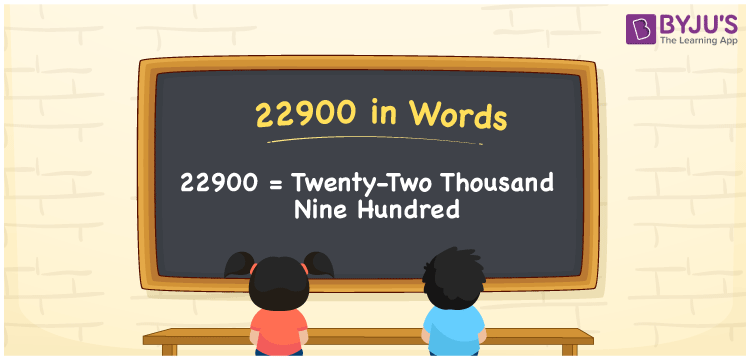# 22900 in words

22900 in words is written as Twenty Two Thousand Nine Hundred. In 22900, the first 2 has a place value of ten thousand, second 2 is in the place value of thousand and 9 is in the place value of hundred. The article on Place Value gives more information. The number 22900 is used in expressions that relate to money, distance, social media views, and many more. For example, “I need to deposit Twenty Two Thousand Nine Hundred Rupees in the bank.”

 22900 in words Twenty Two Thousand Nine Hundred Twenty Two Thousand Nine Hundred in Numbers 22900

## 22900 in English Words## How to Write 22900 in Words?

We can convert 22900 to words using a place value chart. The number 22900 has 5 digits, so let’s make a chart that shows the place value up to 5 digits.

 Ten thousand Thousands Hundreds Tens Ones 2 2 9 0 0

Thus, we can write the expanded form as:

2 × Ten thousand + 2 × Thousand + 9 × Hundred + 0 × Ten + 0 × One

= 2 × 10000 + 2 × 1000 + 9 × 100 + 0 × 10 + 0 × 1

= 22900.

= Twenty Two Thousand Nine Hundred.

22900 is the natural number that is succeeded by 22899 and preceded by 22901.

22900 in words – Twenty Two Thousand Nine Hundred.

Is 22900 an odd number? – No.

Is 22900 an even number? – Yes.

Is 22900 a perfect square number? – No.

Is 22900 a perfect cube number? – No.

Is 22900 a prime number? – No.

Is 22900 a composite number? – Yes.

## Solved Example

1. Write the number 22900 in expanded form

Solution: 2 x 10000 + 2 x 1000 + 9 x 100 + 0 x 10 + 0 x 1

Or Just 2 x 10000 + 2 x 1000 + 9 x 100

We can write 22900 = 20000 + 2000 + 900 + 0 + 0

= 2 x 10000 + 2 x 1000 + 9 x 100 + 0 x 10 + 0 x 1.

## Frequently Asked Questions on 22900 in words

### How to write the number 22900 in words?

22900 in words is written as Twenty Two Thousand Nine Hundred.

### State whether True or False. 22900 is divisible by 3?

False. 22900 is not divisible by 3.

### Is 22900 divisible by 10?

Yes. 22900 is divisible by 10.